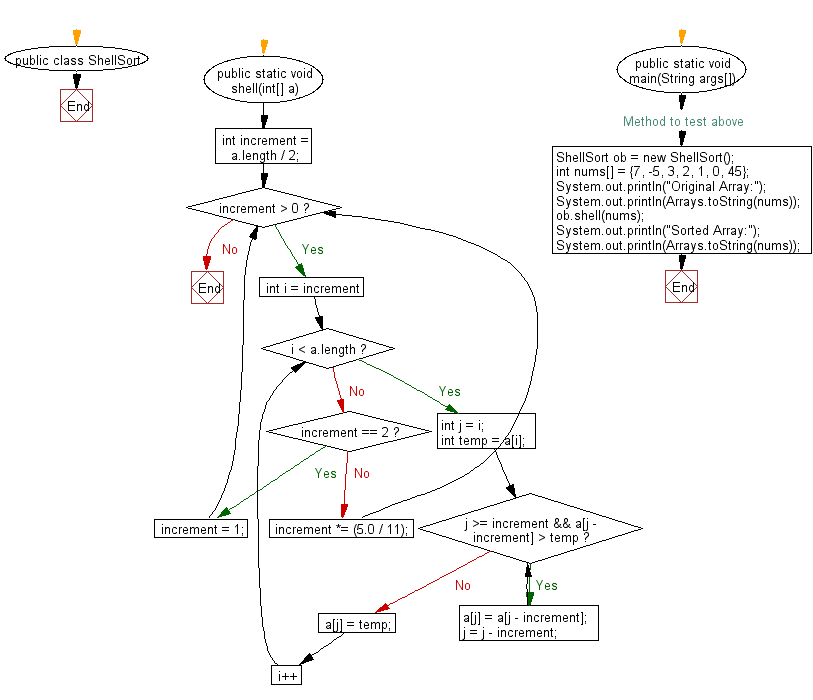﻿ Java exercises: Shell Sort Algorithm - w3resource# Java Exercises: Shell Sort Algorithm

## Java Sorting Algorithm: Exercise-16 with Solution

Write a Java program to sort an array of given integers Shell Sort Algorithm.

According to Wikipedia "Shell sort or Shell's method, is an in-place comparison sort. It can be seen as either a generalization of sorting by exchange (bubble sort) or sorting by insertion (insertion sort). The method starts by sorting pairs of elements far apart from each other, then progressively reducing the gap between elements to be compared. Starting with far apart elements can move some out-of-place elements into position faster than a simple nearest neighbor exchange."

Sample Solution:

Java Code:

``````import java.util.Arrays;
public class ShellSort {
public static void shell(int[] a) {
int increment = a.length / 2;
while (increment > 0) {
for (int i = increment; i < a.length; i++) {
int j = i;
int temp = a[i];
while (j >= increment && a[j - increment] > temp) {
a[j] = a[j - increment];
j = j - increment;
}
a[j] = temp;
}
if (increment == 2) {
increment = 1;
} else {
increment *= (5.0 / 11);
}
}
}

// Method to test above
public static void main(String args[])
{
ShellSort ob = new ShellSort();
int nums[] = {7, -5, 3, 2, 1, 0, 45};
System.out.println("Original Array:");
System.out.println(Arrays.toString(nums));
ob.shell(nums);
System.out.println("Sorted Array:");
System.out.println(Arrays.toString(nums));
}
}
```
```

Sample Output:

```Original Array:
[7, -5, 3, 2, 1, 0, 45]
Sorted Array:
[-5, 0, 1, 2, 3, 7, 45]
```

Flowchart:Java Code Editor:

What is the difficulty level of this exercise?

﻿

## Java: Tips of the Day

Array vs ArrayLists:

The main difference between these two is that an Array is of fixed size so once you have created an Array you cannot change it but the ArrayList is not of fixed size. You can create instances of ArrayLists without specifying its size. So if you create such instances of an ArrayList without specifying its size Java will create an instance of an ArrayList of default size.

Once an ArrayList is full it re-sizes itself. In fact, an ArrayList is internally supported by an array. So when an ArrayList is resized it will slow down its performance a bit as the contents of the old Array must be copied to a new Array.

At the same time, it's compulsory to specify the size of an Array directly or indirectly while creating it. And also Arrays can store both primitives and objects while ArrayLists only can store objects.

Ref: https://bit.ly/3o8L2KH